• 已知不同坐标系的多个坐标点的坐标，求两个坐标系转换矩阵,是matlab写的程序，三维坐标的。 做机器人视觉导航时用到的这个转换。
• 该matlab代码基于武汉大学出版社出版的《大地测量学基础》，为高斯正算程序，代码中详细标注了公式在课本中的位置，以及计算注意事项，可用于多种椭球的大地坐标转换成高斯平面坐标
• 二维平面坐标系转换

千次阅读 2021-04-25 14:26:03
二维平面坐标系转换 在运动控制中，常涉及到物料坐标系与载台坐标系之间的转换。 通常用坐标转换矩阵实现同一点在不同坐标系的转换，即指将两个点之间的仿射关系以转换矩阵的形式表现出来，转换矩阵作用于原始点的...

二维平面坐标系转换

在运动控制中，常涉及到物料坐标系与载台坐标系之间的转换。
通常用坐标转换矩阵实现同一点在不同坐标系的转换，即指将两个点之间的仿射关系以转换矩阵的形式表现出来，转换矩阵作用于原始点的坐标，得到相对应的通过（平移，缩放，旋转）得到的新的点的坐标。

平移：

点P(x,y)平移得到点P'(x',y'),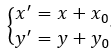矩阵表示：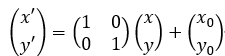缩放：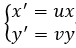矩阵表示：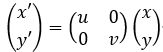旋转：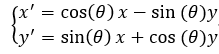矩阵表示：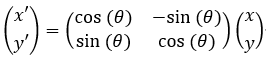旋转证明：

点P向逆时针旋转了θ度，坐标系如下图，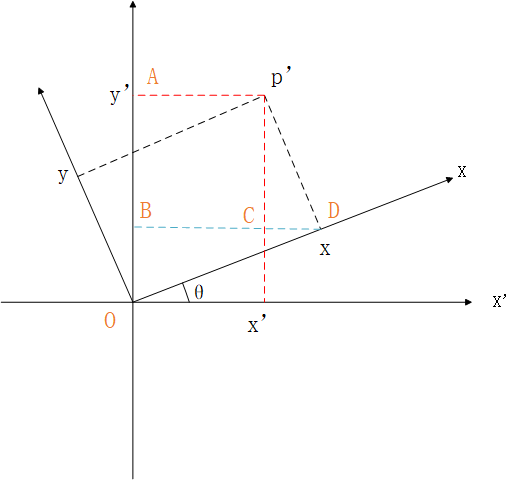依图可知，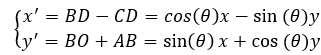其中的旋转角度θ求法：已知对应坐标系下的两点坐标，求两向量的夹角，两向量的夹角的cos值等于两向量的数量积除以两向量的模的乘积

假设，已知物料坐标系中两点坐标p1(x1,y1),p2(x2,y2),其两点在载台坐标系中的坐标为p3(x3,y3),p4(x4,y4),那么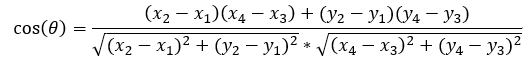坐标转换矩阵

如果一个点同时进行了平移，缩放，旋转，用以下坐标变换矩阵：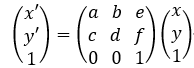即就是a,b,c,d为缩放、旋转的系数，e,f为平移量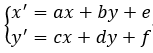eg:
物料坐标系与载台坐标系如下：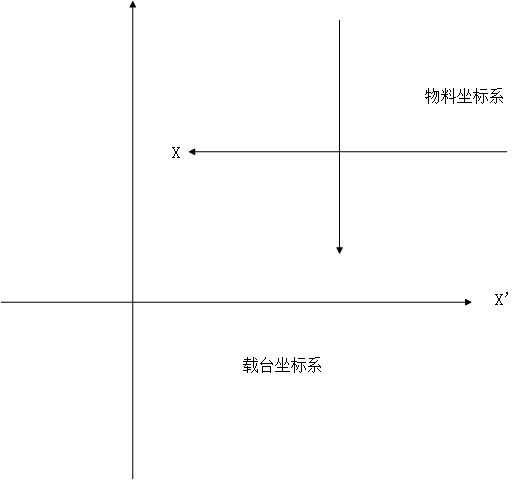先旋转物料坐标系180°，使其与载台坐标系一致：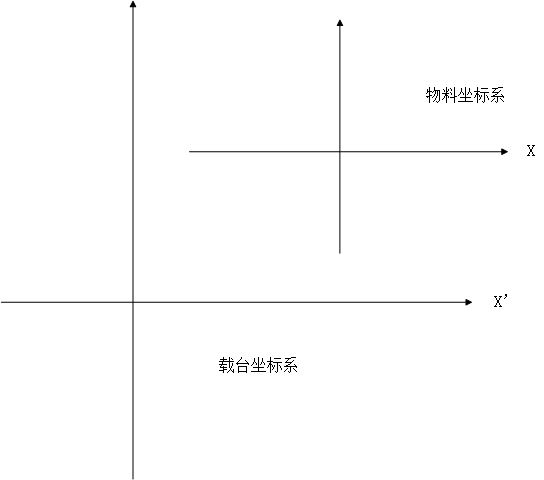C#实现坐标转换矩阵：

using MathNet.Numerics.LinearAlgebra;
using MathNet.Numerics.LinearAlgebra.Double;
class CoorTransform
{
public CoorTransform() { }

public double Mark1_cust_position_x { get; set; }
public double Mark1_cust_position_y { get; set; }
public double Mark2_cust_position_x { get; set; }
public double Mark2_cust_position_y { get; set; }
public double Mark1_stage_position_x { get; set; }
public double Mark1_stage_position_y { get; set; }
public double Mark2_stage_position_x { get; set; }
public double Mark2_stage_position_y { get; set; }
Matrix<double> matrix_rotation { get; set; }   //转换矩阵

// 设置转换矩阵矩阵   如不同坐标系应先完成坐标系转换（这里的不同指左手坐标系和右手坐标系）
public void SetMatrix()
{
double s1_x = Mark2_cust_position_x - Mark1_cust_position_x;
double s1_y = Mark2_cust_position_y - Mark1_cust_position_y;

double s2_x = Mark2_stage_position_x - Mark1_stage_position_x;
double s2_y = Mark2_stage_position_y - Mark1_stage_position_y;

double k = 1;

var vectorA = new DenseVector(new[] { s1_x, s1_y });

var vectorB = new DenseVector(new[] { s2_x, s2_y });

double d = vectorA * vectorB;
//两向量的夹角的cos值=向量的数量积/向量模的乘积    向量的夹角即为两坐标系间的旋转角度
var cos_sg = (vectorA * vectorB) / (Math.Sqrt(s1_x * s1_x + s1_y * s1_y) * System.Math.Sqrt(s2_x * s2_x + s2_y * s2_y));

var sin_sg = Math.Sin(Math.Acos(cos_sg));

//缩放比例
k = Math.Sqrt((s2_x * s2_x + s2_y * s2_y) / (s1_x * s1_x + s1_y * s1_y));

if (((s1_y / s1_x) > (s2_y / s2_x)) & (s1_x > 0))
{
sin_sg = -sin_sg;
}

double[,] stage2 =
{

{ k*cos_sg, k*-sin_sg},
{ k*sin_sg,k*cos_sg},
};

matrix_rotation = DenseMatrix.OfArray(stage2);

//两坐标系间的偏移
double offset_x = Mark1_stage_position_x - Mark1_cust_position_x;
double offset_y = Mark1_stage_position_y - Mark1_cust_position_y;

double[,] stage1 =
{
{ k*cos_sg, k*-sin_sg,offset_x},
{ k*sin_sg,k*cos_sg,offset_y},
{ 0,0,1}
};

matrix_rotation = DenseMatrix.OfArray(stage1);
}

public void Check(double x1, double y1, double x2, double y2, double x3, double y3, double x4, double y4,double x5, double y5)
{
Mark1_cust_position_x = x1;
Mark1_cust_position_y = y1;
Mark2_cust_position_x = x2;
Mark2_cust_position_y = y2;
Mark1_stage_position_x = x3;
Mark1_stage_position_y = y3;
Mark2_stage_position_x = x4;
Mark2_stage_position_y = y4;

SetMatrix();
//由于物料坐标系做了旋转变换(180°)，故此坐标点均乘以-1，即旋转矩阵（-1  0
//                                                           0  -1)
var vectorsssss = new DenseVector(new[] { -x5, -y5, 1 });
var result = matrix_rotation * vectorsssss;
}
}

展开全文坐标系转换 转换矩阵
• 空间坐标系以及空间两三维坐标系转换矩阵解释 因为工作的原因，需要进行不同坐标系的一个转换，在查阅了众多的网页之后，发现有用的没几个，而且众多都是抄的同一篇文章，而且都是罗列以下旋转的方式，几乎没有解释...

空间坐标系以及空间两三维坐标系转换矩阵解释

因为工作的原因，需要进行不同坐标系的一个转换，在查阅了众多的网页之后，发现有用的没几个，而且众多都是抄的同一篇文章，而且都是罗列以下旋转的方式，几乎没有解释旋转角的物理意义，也不说这个旋转角怎么得到的。在折腾了一天之后，终于弄清楚了，特意写下来，既能有助于记忆，又能装b，很好很强大。

基础背景

空间三维坐标系转换，大多出现在像我似的搞地图的这群B人里，当然还有搞游戏的大佬们。所以需要对空间三维坐标系做一个小的总结，来防止忘记或者混乱。

具体图形可百度，赖的放图

• 地心坐标系
空间三维坐标系，左手系
• 高斯坐标系
在搞激光点云的人眼里，基本上就指的是，当前度带（分3度带和6度带）中的高斯直角坐标系，右手系，点云中记录的点坐标大多有经纬度坐标、高斯坐标，两种坐标。
• 像空间坐标系
z轴正方向，与主光轴方向重合，x、y轴方向与相平面坐标系平行。右手系
• 像平面坐标系
xy轴方向与像素坐标系平行，单位为m，是一个物理坐标系
• 像素坐标
单位为像素，在与像平面坐标系进行转换的时候，需要知道每个像素的物理尺寸

三维坐标系转换

为了通用性，前边的空间坐标系只做一个概念上的罗列，这里针对任意两空间直角坐标系的相互转换进行解释。

• 世界坐标系XYZ：仅仅用于表述，不代表物理意义上世界坐标系
• 局部坐标系xyz
• 旋转角：重点需要搞清楚，这个旋转角是从哪个坐标系旋转到哪个坐标系，在地图行业里的多传感器的联合标定中，这个旋转角是由相应的标定文件提供的。

已知条件

1. 世界坐标系的三个坐标轴：world_x（1，0，0），world_y(0, 1, 0)，world_z（0，0，1）
2. 局部坐标系的三个坐标轴：local_x（g，h，i），local_y（d，e，f）local_z（a，b，c）
其中，局部坐标系的坐标轴坐标均为世界坐标系下的坐标值，这点非常重要，同时，两空间三维坐标系还有个平移向量，因为只是很简单的坐标值平移，这里就不再赘述，默认两坐标系的原点相同。

旋转原理

这里盗一张图（找了好久，看到的一个良心博客 http://www.skcircle.com/?id=515）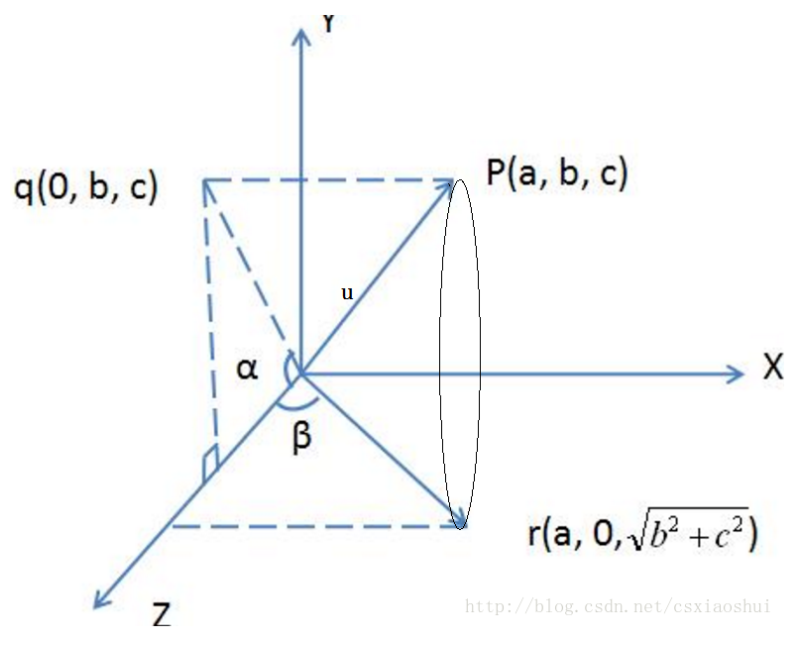如上图，P点为局部坐标系的z轴上一点，q为点P在世界坐标系ZOY平面上的投影点，r为点P在经过旋转之后的对应点，如果你去查看了贴的那个网页博客，请不要看他的旋转方式，并不是大佬描述错误，而是不适用空间三维坐标系的相互转换，因为这里涉及到一个很蛋疼的说法，这个旋转矩阵描述的是A坐标系到B坐标系转换方式

世界坐标系到局部坐标系的旋转矩阵R

注意：XYZ与xyz，我都会严格注意，不会写错！（写错老子就改！看其他博客的时候，就深受其害），为了描述方便，世界坐标系的轴或者坐标都会用大写的XYZ来代替，局部坐标用xyz来代替，而且为了防止0与O不好分辨，所有的0都用汉字零来表示

问：如何理解旋转矩阵究竟是描述的A到B，还是B到A？

答：旋转世界坐标系，使得世界坐标系与局部坐标系重合，那么得到的这个旋转矩阵，描述的是，世界坐标系到局部坐标系转换关系，用公式来表达

local = R * world

结合上方图片：（注意理解旋转角）

1. 逆时针旋转世界坐标系X轴，使得局部坐标系的 z轴 与世界坐标系的 XOZ 平面共面，结合上图（那个椭圆就是为了方便理解，而虚拟出来的点P的旋转轨迹），要想OP出现在XOZ平面上，就需要逆时针旋转X轴，旋转的角度为θ（注意，α为向量夹角，范围0 - 180，如果这个角不会求，要不，再去从小学开始复读一下吧）。但是注意，旋转角是有方向的，图里的例子，如果要逆时针把OZ，旋转到Oq，那么旋转的角度应该是360 - α。

注意：其实顺时针也可以，只需要保证所有的轴都同时为逆时针或者顺时针即可，不过大家都通用逆时针而已，所以，请把顺时针给从脑子里删除

• 如何来获取旋转角度θ（而非向量夹角）
就需要用到向量的叉乘，来确定向量的左右侧。这里要将OZ旋转到Oq，那么OZ就是基准，需要知道Oq在OZ的左侧还是右侧，图里是在右侧，与逆时针相反，用叉乘表示： OZ ✖ Oq 得到的向量与X轴的乘积小于零，那么旋转角度就应该是θ = 360 - α，如果在OZ的左侧的话，向量夹角就是旋转角度，θ = α。

旋转的是X轴，那么矩阵描述应该为Rx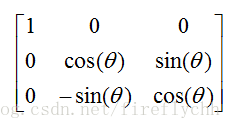1. 经过步骤1之后，局部坐标系的z轴现在就表现为图里的Or，接着逆时针旋转世界坐标系的Y轴，使得世界坐标系的Z轴与局部坐标系得z轴重合，同理，观察Or在OZ得左侧，那么这一步得旋转角度θ = β。
旋转的是轴，那么矩阵描述应该为Ry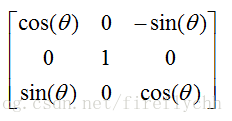2. 经过前两步得旋转，此时世界坐标系得Z轴与局部坐标系得z轴已经重合了，那么接着逆时针旋转世界坐标系Z轴，就是一个简单得二维上得旋转了，就会很好理解了，如下图所示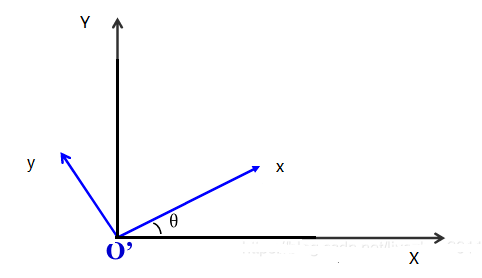同理，求出旋转角θ，这次旋转的是世界坐标系的Z轴，那么旋转矩阵就是Rz，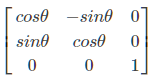结束
经过上边的三步旋转，就将世界坐标系，旋转到了局部坐标系上，通过矩阵的左乘，就能得到世界坐标系到局部坐标系的旋转矩阵R
R = Rz * Ry * Rx

完整坐标值形式（加上偏移值）
local = R * world + T
其中T为世界坐标系原点在 局部坐标系中的坐标

局部坐标系到世界坐标系的旋转矩阵

但是现实中，往往是知道局部坐标系原点在世界坐标系中的坐标，因此，基本上都是求得局部坐标系到世界坐标系得旋转矩阵（上边的R，求个逆即可，自己玩去吧您那，赖的写了）
2021.11.12号，补充
还是写清楚吧，方便各位跟我一样的小白。
上边的旋转矩阵R，描述的是world到local的旋转方式，那么将R求逆得到W，同时知道局部坐标系原点在世界坐标系中的坐标O，那么local坐标转到world坐标的方式如下

world = W * local + O

展开全文3d 线性代数
• 二、平面坐标变换矩阵 三、平移变换 四、比例变换 五、对称变换 六、旋转变换 七、错切变换 八、复合变换 (1)、复合平移 （2）复合比例变换 （3）复合旋转 （4）相对某点的比例变换 （5）相对某点的选址...

目录

一、平面直角坐标系的建立

二、平面坐标变换矩阵

三、平移变换

四、比例变换

五、对称变换

六、旋转变换

七、错切变换

八、复合变换

(1)、复合平移

（2）复合比例变换

（3）复合旋转

（4）相对某点的比例变换

（5）相对某点的选址变换

po一个B站线性代数学习资料，这个作者很好地解释线性代数操作空间的本质。

【官方双语/合集】线性代数的本质 - 系列合集

一、平面直角坐标系的建立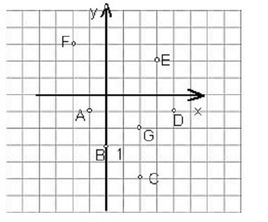在平面上选一点作为直角坐标的原点，过该原点作相互垂直的两轴，就建立起了平面直角坐标系，如上图所示。

在代码中，我们可以用一个类表示一个点实体，他由一串坐标组成，但是，如果这些点如果位于不同的坐标系中，该怎么转换呢？通过对X,Y的操作，比如平移就在相应的X,Y分量上加偏移量，我们就可以实现。那如果，我们既要平移，又要旋转，或者一系列的对点实体的操作，该怎么实现？这个时候就可以用到平面坐标变换矩阵。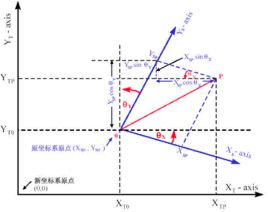二、平面坐标变换矩阵

“变换矩阵是数学线性代数中的一个概念。在线性代数中，线性变换能够用矩阵表示。如果T是一个把Rn映射到Rm的线性变换，且x是一个具有n个元素的列向量 ，那么我们把m×n的矩阵A，称为T的变换矩阵。”

-------百度百科-变换矩阵

其实没有这么复杂，就是我们通过对一个坐标串构成的矩阵与某个矩阵相乘，得到的新矩阵包含了我们所要的坐标的信息。这个"某个矩阵"在这里就是屏幕坐标变换矩阵。

怎么构建 矩阵吧，矩阵的构建可以用二维数组实现。这个不是算法的重点，所以我就不po代码了，想看代码可以到我的github上看

https://github.com/XiaoZhong233/GIS_ALG/blob/master/src/scau/gz/zhw/BasicTransform.java

平面坐标变换矩阵可由下式表示：

/**
*      |a d g|
* T= |b e h|      |a d|                                                                                                             |g|
*       |c f   i|      |b e| 负责对图形的缩放,旋转,对称,错切 。[c f] 负责对图形进行平移变换    |h| 负责投影变换
*/

构建代码：

public class SurfaceTransformationMatrix {

private double a,b,c,d,e,f,g,h,i;
private double[][]  data= {{a,d,g},{b,e,h},{c,f,i}};
private Matrix matrix;

public SurfaceTransformationMatrix() {
this.matrix = new Matrix(data);
}

public SurfaceTransformationMatrix(double[][] data) {
this.matrix = new Matrix(data);
}

public Matrix getMatrix() {
return matrix;
}

}

三、平移变换

公式如下：(m,n)是变换后的坐标，(x,y)是变换前的坐标，tx,ty分别对应x轴，y轴的偏移量

构建代码：

public class TransformMatrix extends SurfaceTransformationMatrix{

private Matrix matrix;

public TransformMatrix(double Tx,double Ty) {
super(new double[][]{{1,0,0},{0,1,0},{Tx,Ty,1}});
this.matrix = super.getMatrix();
}

public Matrix getTransformMatrix() {
return matrix;
}

}

平移算法：

/**
* 平移算法
* @param point
* @param x	x正方向偏移量
* @param y	y正方向偏移量
* @return
*/
public static Point transform(Point point,double x,double y) {
Matrix matrix = new TransformMatrix(x, y).getTransformMatrix();
double [][] data= {{point.getX(),point.getY(),1}};
Matrix pointMatrix = new Matrix(data);
Matrix result = pointMatrix.RightMultiMatrix(matrix);
//System.out.println("平移后的点 ："+new Point(result.getMatrix(), result.getMatrix()).toString());
return new Point(result.getMatrix(), result.getMatrix());
}

public static Line transform(Line line,double x,double y) {
Point start = line.getStart();
Point end = line.getEnd();

Point newStart = transform(start, x, y);
Point newEnd = transform(end, x, y);
return new Line(newStart,newEnd);
}

public static Polygon transform(Polygon polygon,double x,double y) {
Point[] points = polygon.getPoints();
Point[] result = new Point[points.length];
for(int i=0;i<points.length;i++) {
result[i]=transform(points[i], x, y);
//System.out.println("result ："+result[i].toString());
}
return new Polygon(result, polygon.isClose());
}

四、比例变换

变换公式：[x* y* 1] = [x y 1] x [{Sx,0,0},{0,Sy,0},{0,0,1}] = [Sx*x Sy*y 1]

因为公式没找到图，就用二维数组来表示

x*,y*是x,y变换后的坐标

变换关系如下

（1）当Sx = Sy = 1 时，为恒等比例变换，就是图形不变

（2）当Sx = Sy > 1 时，图形沿两个坐标轴方向等比例放大。

（3）当Sx = Sy < 1 时，图形沿两个坐标轴方向等比例缩小。

（4）当Sx != Sy  时，图形沿两个坐标轴方向做非均匀的比例变换。

构建代码：

public class ScaleMtrix extends SurfaceTransformationMatrix{
private Matrix matrix;
public ScaleMtrix(double Sx,double Sy) {
// TODO Auto-generated constructor stub
super(new double[][]{{Sx,0,0},{0,Sy,0},{0,0,1}});
this.matrix = super.getMatrix();
}

public Matrix getScaleMatrix() {
return matrix;
}
}

算法：

/**
* 比例变换算法
* x=y时，恒比例放大或缩小
* x!=y时，图形沿两个坐标轴方向做非均匀比例变换
* @param point
* @param x
* @param y
* @return
*/
public static Point scale(Point point,double x,double y) {
Matrix matrix = new ScaleMtrix(x, y).getScaleMatrix();
double [][] data= {{point.getX(),point.getY(),1}};
Matrix pointMatrix = new Matrix(data);
Matrix result = pointMatrix.RightMultiMatrix(matrix);
return new Point(result.getMatrix(), result.getMatrix());
}

public static Line scale(Line line,double x,double y) {
Point start = line.getStart();
Point end = line.getEnd();

Point newStart = scale(start, x, y);
Point newEnd = scale(end, x, y);
return new Line(newStart,newEnd);
}

public static Polygon scale(Polygon polygon,double x,double y) {
Point[] points = polygon.getPoints();
Point[] result = new Point[points.length];
for(int i=0;i<points.length;i++) {
result[i]=scale(points[i], x, y);
//System.out.println("result ："+result[i].toString());
}
return new Polygon(result, polygon.isClose());
}

五、对称变换

公式如下：

[x*,y*,1] = [x,y,1] x [{a,d,0},{b,e,0},{0,0,1}] = [ax+by dx+ey 1]

变换关系：

（1）当b=d=0,a=-1,e=1时，产生与y轴对称的反射图形

（2）当b=d=0,a=1,e=-1时，产生与x轴对称的反射图形

（3）当b=d=0,a=e=-1时，产生与原点对称的反射图形

（4）当b=d=1,a=e=0时，产生与直线y=x对称的反射图形

（5）当b=d=-1,a=e=0时，产生与直线y=-x对称的反射图形

构建代码：

/**
* 对称变换矩阵
*
*/
public class SymmetryMatrix extends SurfaceTransformationMatrix{
private Matrix matrix;

public SymmetryMatrix(double a,double b,double d,double e) {
// TODO Auto-generated constructor stub
super(new double[][] {{a,d,0},{b,e,0},{0,0,1}});
this.matrix = super.getMatrix();
}

public Matrix getSymmetryMatrix() {
return matrix;
}
}

算法：

/**
* 对称变换
* @param point
* @param symmetryType 枚举类型
* @return
*/
public static Point symmetry(Point point,SymmetryType symmetryType) {
Matrix matrix;
switch (symmetryType) {
case xAxis:
matrix = new SymmetryMatrix(1, 0, 0, -1).getSymmetryMatrix();
break;
case yAxis:
matrix = new SymmetryMatrix(-1, 0, 0, 1).getSymmetryMatrix();
break;
case yx:
matrix = new SymmetryMatrix(0, 1, 1, 0).getSymmetryMatrix();
break;
case anti_yx:
matrix = new SymmetryMatrix(0, -1, -1, 0).getSymmetryMatrix();
break;
case origin:
matrix = new SymmetryMatrix(-1, 0, 0, -1).getSymmetryMatrix();
default:
matrix = new SymmetryMatrix(-1, 0, 0, -1).getSymmetryMatrix();
break;
}
double [][] data= {{point.getX(),point.getY(),1}};
Matrix pointMatrix = new Matrix(data);
Matrix result = pointMatrix.RightMultiMatrix(matrix);
return new Point(result.getMatrix(), result.getMatrix());
}

六、旋转变换

公式如下：

[x,y,1] x [{cosa,sina,0},{-sina,cosa,0},{0,0,1}] = [xcosa-ysina xsina+ycosa 1]

a是二维图形绕原点顺时针旋转a角。

构建代码：

public class RotateMatrix extends SurfaceTransformationMatrix{

private Matrix matrix;

public RotateMatrix(double angle) {
// TODO Auto-generated constructor stub
{0,0,1}});
this.matrix = super.getMatrix();
}

public Matrix getRotateMatrix() {
return matrix;
}
}

算法：

/**
* 旋转变换
* @param point
* @param angle 角度制单位
* @return
*/
public static Point rotate(Point point,double angle) {
Matrix matrix = new RotateMatrix(angle).getRotateMatrix();
double [][] data= {{point.getX(),point.getY(),1}};
Matrix pointMatrix = new Matrix(data);
Matrix result = pointMatrix.RightMultiMatrix(matrix);
return new Point(result.getMatrix(), result.getMatrix());
}

七、错切变换

公式如下：

[x*,y*,1] = [x,y,1] * [{1,d,0},{b,1,0},{0,0,1}]  = [x+by,dx+y,1]

x*,y*为变换后的坐标。

变换关系如下：

（1）当d=0时，x*=x+by,y*=y,此时图形的y坐标不变，x坐标随初值(x,y)及变换系数b而作线性变换；若b>0，则图形沿+x方向做错切位移；b<0图形沿-x方向做错切位移。

（2）当b=0时，x*=x,y*=dx+y，此时图形的x坐标不变，y坐标随初值（x,y）及变换系数d做线性变换；如d>0，则图形沿+y方向作错切变换；d<0时，图形沿-y方向做错切位移。

（3）当b!=0时，且d!=0时，x*=x+by，y*=dx+y，图形沿x,y两个方向错切位移。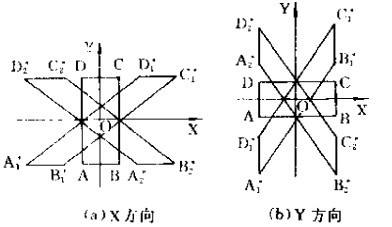构建代码：

public class MiscutMatrix extends SurfaceTransformationMatrix{
private Matrix matrix;

public MiscutMatrix(double d,double b) {
// TODO Auto-generated constructor stub
super(new double[][] {{1,d,0},{b,1,0},{0,0,1}});
this.matrix = super.getMatrix();
}

public Matrix getMiscutMatrix(){
return matrix;
}
}

算法如下：

/**
* 错切变换
* @param point
* @param b=0,y轴随变换系数d变换  b>0,图形沿+y方向做错切变换,b<0,图形沿-y方向做错切变换
* @param d=0,y轴随变换系数b变换  b>0,图形沿+x方向做错切变换,b<0,图形沿-x方向做错切变换
* 		  b!=0 && d!=0时,x*=x+by y*=dx+y 图形沿x,y两个方向做错切变换
* @return
*/
public static Point miscut(Point point,double b,double d) {
Matrix matrix = new MiscutMatrix(b,d).getMiscutMatrix();
double [][] data= {{point.getX(),point.getY(),1}};
Matrix pointMatrix = new Matrix(data);
Matrix result = pointMatrix.RightMultiMatrix(matrix);
return new Point(result.getMatrix(), result.getMatrix());
}

八、复合变换

复合变换是指图形做一次以上的几何变换，变换结果是每次变换矩阵相乘。

(1)、复合平移

直接上代码吧，就直接几个平移矩阵相乘

/**
* 复合平移
* @param point
* @param matrixs
* @return
*/
public static Point complexTransform(Point point,TransformMatrix...matrixs) {
int len = matrixs.length;
Matrix matrix = matrixs.getTransformMatrix();

for(int i=1;i<len;i++) {
matrix = matrix.RightMultiMatrix(matrixs[i].getTransformMatrix());
}
double [][] data= {{point.getX(),point.getY(),1}};
Matrix pointMatrix = new Matrix(data);
Matrix result = pointMatrix.RightMultiMatrix(matrix);
return new Point(result.getMatrix(), result.getMatrix());
}

（2）复合比例变换

/**
* 复合比例变换
* @param point
* @param matrixs
* @return
*/
public static Point complexScale(Point point,ScaleMtrix...matrixs) {
int len = matrixs.length;
Matrix matrix = matrixs.getScaleMatrix();
for(int i=1;i<len;i++) {
matrix = matrix.RightMultiMatrix(matrixs[i].getScaleMatrix());
}
double [][] data= {{point.getX(),point.getY(),1}};
Matrix pointMatrix = new Matrix(data);
Matrix result = pointMatrix.RightMultiMatrix(matrix);
return new Point(result.getMatrix(), result.getMatrix());
}

（3）复合旋转

/**
* 复合旋转变换
* @param point
* @param matrixs
* @return
*/
public static Point complexRotate(Point point,RotateMatrix ...matrixs) {
int len = matrixs.length;
Matrix matrix = matrixs.getRotateMatrix();
for(int i=1;i<len;i++) {
matrix = matrix.RightMultiMatrix(matrixs[i].getRotateMatrix());
}
double [][] data= {{point.getX(),point.getY(),1}};
Matrix pointMatrix = new Matrix(data);
Matrix result = pointMatrix.RightMultiMatrix(matrix);
return new Point(result.getMatrix(), result.getMatrix());
}

比例，旋转变换是与参考的有关的，上面的都是相对于原点的比例变换，如果要参考某个(m,n)点做比例 ，旋转变换，其变换过程就是先把该坐标系的原点移到（m,n）上来，然后做了旋转或比例变换，然后再移回去。

（4）相对某点的比例变换

/**
* 相对于某点的比例变换
* @param point 待变换的点
* @param center 相对点
* @param x
* @param y
* @return
*/
public static Point scaleAround(Point point,Point center,double x,double y) {
TransformMatrix t1 = new TransformMatrix(-center.getX(), -center.getY());
ScaleMtrix scaleMtrix = new ScaleMtrix(x, y);
TransformMatrix t2 = new TransformMatrix(center.getX(), center.getY());
return complexTransmit(point, t1,scaleMtrix,t2);
}

（5）相对某点的选址变换

/**
* 围绕某点的旋转变换
* @param point 待变换的点
* @param center 相对点
* @param angle
* @return
*/
public static Point rotateAround(Point point,Point center,double angle) {
TransformMatrix t1 = new TransformMatrix(-center.getX(), -center.getY());
RotateMatrix rotateMatrix = new RotateMatrix(angle);
TransformMatrix t2 = new TransformMatrix(center.getX(), center.getY());
return complexTransmit(point, t1,rotateMatrix,t2);
}

最后 po上我github地址，有需要的同学可以看看

https://github.com/XiaoZhong233/GIS_ALG/blob/master/src/scau/gz/zhw/BasicTransform.java

展开全文JAVA GIS
• 批量点，矩阵形式给出，MATLAB经纬度坐标转换平面坐标；Mercator投影变换。调用单点经纬度坐标转换平面坐标的函数也在文件中。博客中有详细解释和C++代码...
• 1.像素坐标系、像平面坐标希、相机坐标系、世界坐标系的定义；2.四个坐标系之间的相互转换关系

像素坐标系

以图像左上角为原点建立以像素为单位的直接坐标系 u − v u-v 。横轴为 u u ，向右为正方向；纵轴为 v v ，向下为正方向
u u v v 代表像素的行数和列数，没有物理单位像平面坐标系

像平面坐标系 o − x y o-xy 是以影像几何中心 o o 为原点， x x y y 轴方向分别为平行于影像画幅边缘线的二维坐标系相机坐标系

相机坐标系 o c − x c y c z c o_c-x_cy_cz_c 是以摄像机光心为原点（在针孔模型中也就是针孔为光心），z轴与光轴重合也就是z轴指向相机的前方（也就是与成像平面垂直），x轴与y轴的正方向与像平面坐标系平行的三维直角坐标系

注意下图不符合针孔成像，只是示意图。如果是针孔成像，z轴方向与下图相反世界坐标系

由于摄像机可安放在环境中的任意位置，在环境中选择一个基准坐标系来描述摄像机的位置，并用它描述环境中任何物体的位置，该坐标系称为世界坐标系 o w − x w y w z w o_w-x_wy_wz_w
相机坐标系与世界坐标系之间的关系可以用旋转矩阵与平移向量来描述

像素坐标系<=>像平面坐标系

假设 ( u 0 , v 0 ) (u_0,v_0) 代表 O 1 O_1 u − v u-v 坐标系下的坐标， d x d_x d y d_y 分别表示每个像素在像平面坐标系横轴 x x 和纵轴 y y 上的物理尺寸
则像素坐标系和像平面坐标系有如下关系：写成矩阵形式，就是：像素坐标系<=>相机坐标系更正：
上图中红色的不是像平面坐标系，而是原点从左上平移到中心的像素坐标系！

写成矩阵形式，就是：相机坐标系<=>世界坐标系

世界坐标系是为了描述相机的位置而被引入的
平移向量 t t 和旋转矩阵 R R 可以用来表示相机坐标系与世界坐标系的关系
所以，假设空间点 P P 在世界坐标系下的齐次坐标是 ( x w , y w , z w , 1 ) T (x_w, y_w, z_w, 1)^T ，在相机坐标系下的齐次坐标是 ( x c , y c , z c , 1 ) T (x_c, y_c, z_c, 1)^T ，则存在如下关系：结语

如果您有修改意见或问题，欢迎留言或者通过邮箱和我联系。
手打很辛苦，如果我的文章对您有帮助，转载请注明出处。

展开全文• 不同坐标系的矩阵变换

万次阅读 2018-03-24 20:14:18
转载自：https://blog.csdn.net/jc_laoshu/article/details/69657579概要：三维坐标系的变换，实质上则是原点以及正交基向量的变化，在空间中表现为平移和旋转。如图所示的坐标系变换，可以用一个变换矩阵来...
• 坐标系旋转矩阵推导过程

千次阅读 2018-09-04 14:42:08
一、先来个平面旋转的分析：     两角和(差)公式 推导 旋转变换一般是按照某个圆心点，以一定半径 r 旋转一定的角度α，为了简单起见我们给出下面的情景 假定点A(x,y)想经过旋转变换到达B(x',y')，已知...
• 坐标转换矩阵 什么是坐标转换矩阵 坐标转换矩阵就是指将两个点之间的仿射关系以转换矩阵的形式表现出来，也就是通过转换矩阵作用于原始点的坐标，得到 ...变换矩阵 坐标 旋转矩阵
• 根据 三点在两个坐标系下的坐标，建立两个坐标系之间的旋转矩阵R和平移向量T，适用场景：求 世界坐标系到 相机坐标系转换关系。以其中一点建立 世界坐标系，该点在相机坐标系中的坐标是 世界坐标系坐标系的平移...计算机视觉
• #Android 的坐标系矩阵变换##Android的坐标系##2D坐标系android的2d坐标系如图所示，水平向右为X轴正方向，竖直向下为Y轴正方向，原点为屏幕左上角。 注意：以屏幕左上角为原点的坐标系，称作绝对坐标系，将原点平...
• 之前整理过：《透析矩阵，由浅入深娓娓道来—高数-线性代数-矩阵》、《三维旋转笔记:欧拉角/四元数/旋转矩阵/轴角-记忆点整理》，这次转载FuckGIS的《Cesium之球心坐标与本地坐标》，算是线性代数在前端领域的的又一...Cesium 矩阵变换 球心坐标 本地坐标系
• 本篇讨论的主题是在平面坐标系中如何将一个坐标系（目标坐标系，以下简称目标系）中的所有点投射到另一个坐标系（基坐标系）中。平面坐标系之间的转化一般有三步操作：1、平移；2、旋转；3、拉伸。在转化的过程中...坐标系转化 平面坐标系 c# math
• OpenGL坐标转换采用的是局部坐标系，其特点有一下几点： ①每一次转换相对于自身坐标系 ②转换叠加采用右乘矩阵的方式 ③后调用先执行 全局坐标系与之相反，故在进行转换时所用的转换函数有所不同。 本文利用...OpenGL 矩阵转换
• 平面坐标系变换

千次阅读 2018-11-14 18:44:38
平面坐标系变换 flyfish x′=xcos⁡θ+ysin⁡θx&amp;#x27; = x \cos \theta + y \sin \theta x′=xcosθ+ysinθ y′=−xsin⁡θ+ycos⁡θy&amp;#x27; = - x \sin \theta + y \cos \theta y′=−xsinθ+ycos...
• 最近在网上看到了很多关于坐标系转换的帖子，但是其内容很多都是相互转载（甚至还有一部分是错误的），同时大部分的文章内容都是告诉你四种坐标系间的相互转化的数学公式，看完之后很多时候还是不知所云，本文意在...相机坐标系 图像像素坐标系 图像物理坐标系 世界坐标系
• 1)比例变换比例变换就是将平面上任意一点的横坐标放大或缩小S11倍，纵坐标放大或缩小S22倍，即其中S称为比例变换矩阵。图2．24是比例变换的几个例子。图中(b)是S11=S22的情况，(C)是S11≠S21的情况2)旋转变换旋转...
• 平面坐标变换 矩阵形式

千次阅读 2014-09-27 21:36:26
﻿﻿ ... 2.3.3 基本二维变换  基本二维变换有比例变换（Scaling）、旋转变换（Rotating）、错切变换（Shearing）和平移变换（Translating）。...比例变换就是将平面上任意一点的横坐标放大或缩小S11
• 主要分析从局部坐标系变换到全局坐标系下的坐标转换公式。首先对全局坐标系进行描述，如图1，o-xyz即全局坐标系，O’-X’Y’Z’即在o-xyz坐标系中定义的局部坐标系，两个坐标系之间的关系如下：这个O’-X’Y’Z’的Z...坐标系变换 全局坐标系 局部坐标系
• 四参数的转换公式为： 根据最小二乘原理，构造条件方程： 设a = mcosa-1, b=msina,有: 等价表达： 其中，，, 解得X后，求m和α：
• 世界坐标系、相机坐标系、图像平面坐标系

万次阅读 多人点赞 2018-07-12 18:04:09
一、四个坐标系简介和转换 相机模型为以后一切标定算法的关键，只有这边有相当透彻的理解，对以后的标定算法才能有更好的理解。...我觉得首先我们要理解相机模型中的四个平面坐标系的关系：像素平面坐标系（u,v...
• 本文主要用于回顾二维平面上点的转换矩阵相关概念和应用。 1. 平移 （Translation） 在2D空间中，我们经常需要将一个点平移到另一个位置。假设空间中的一点P，其用坐标表示为（x,y）；将其向 x方向平移 tx，向y方向...
• 图像坐标系、相机坐标系和世界坐标系的定义，及三者之间的变换关系。 图像坐标系 1.图像像素坐标系 原点：图像左上角P0点 单位：像素 横坐标u：图像数组中的列数 纵坐标v：图像数组中的行数 2.图像物理坐标系 在...图像坐标系 相机坐标系 世界坐标系 相机内外参数矩阵
• 目录一、各坐标系简要介绍二、坐标系转换2.1 世界坐标系转为相机坐标系2.2 相机坐标系转为物理图像坐标系2.3 物理图像坐标系转为图像坐标系2.4 总结2.5 为什么要使用齐次坐标参考文献 一、各坐标系简要介绍 首先...计算机视觉 人工智能
• 实际的坐标系转换应用中，一般是要得到测量坐标系和装配坐标系的关系。在这种情况下，如果使用原来的方法，点在装配坐标系的位置测量需要使用其他方法。本文介绍一种不需要知道装配坐标系中点的具体坐标就可以进行...SVD平面拟合 算法 代码
• 关节坐标系和世界坐标系是工业机器人的两个基本坐标系，两者之间相互转换的算法是机器人算法的基础。各机器人厂家有各自的算法，但不会向使用者详细介绍，所以机器人使用者往往不易更加深入了解机器人的内部算法，这...
• 坐标系转换分析

万次阅读 多人点赞 2018-01-31 10:41:05
二维平面直角坐标系定义可分为两类，从逆时针角度看，第一类为X坐标轴在Y坐标轴后；第二类为X坐标轴在Y坐标轴前。 有这两类坐标系添加第三个坐标轴Z,得到空间直角坐标系，在默认添加的坐标轴Z垂直纸面朝向外侧下，......